# Econometrics ch11

8 Sep 2016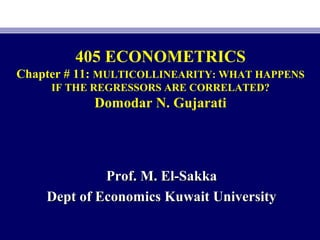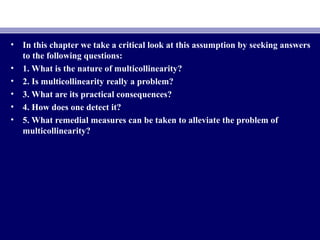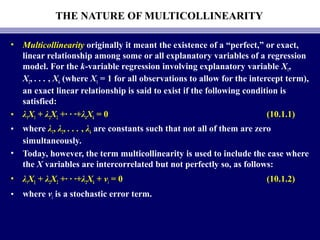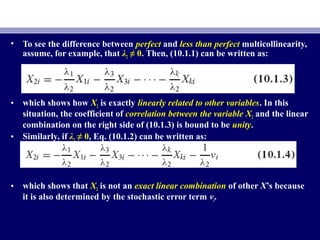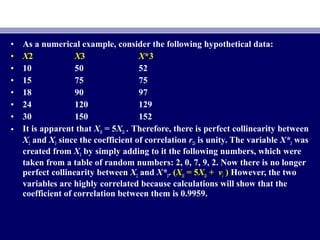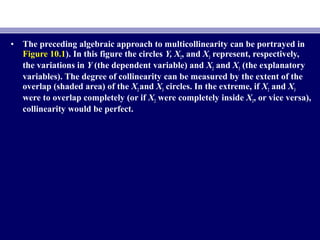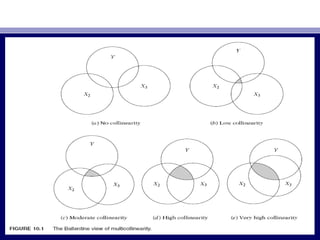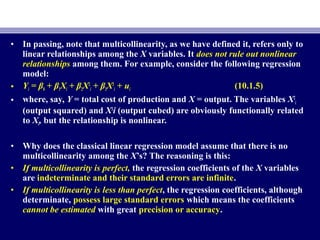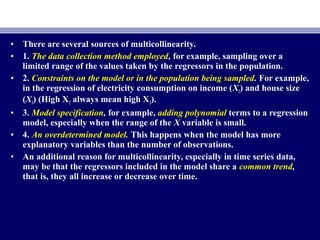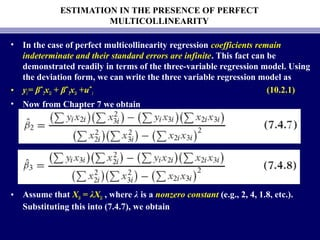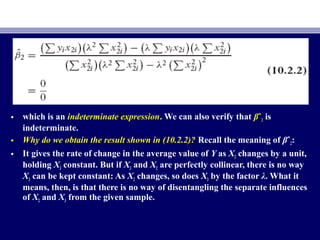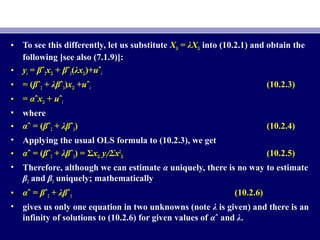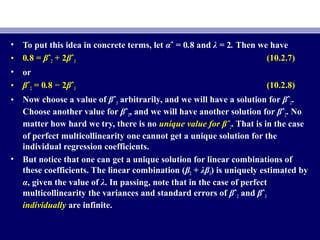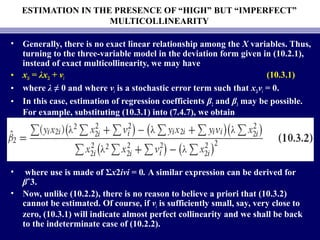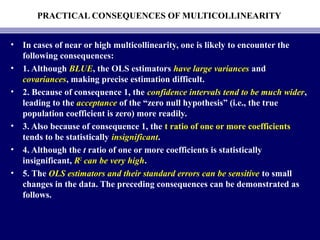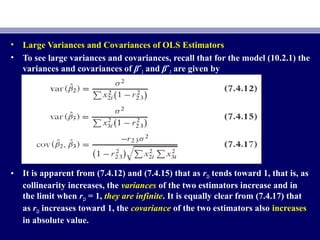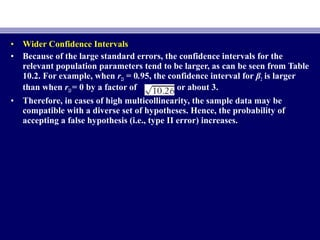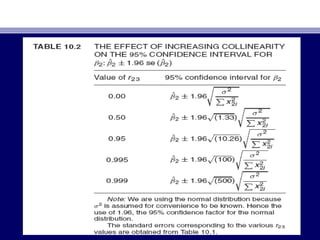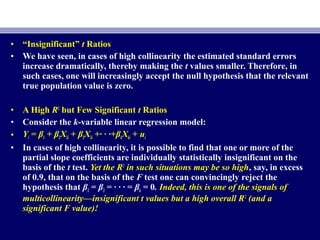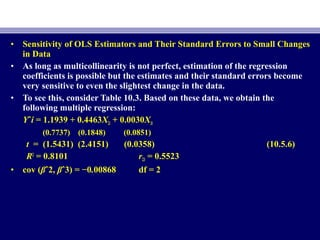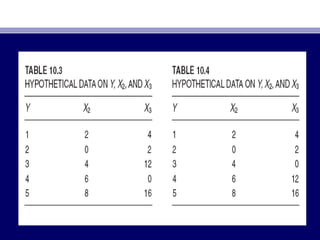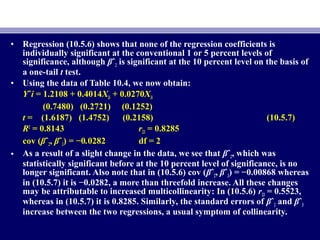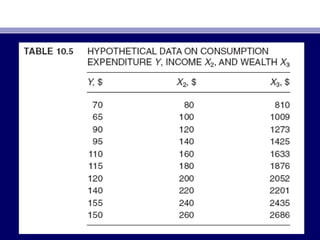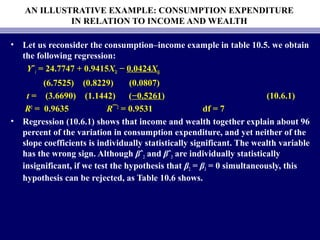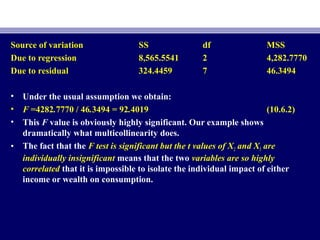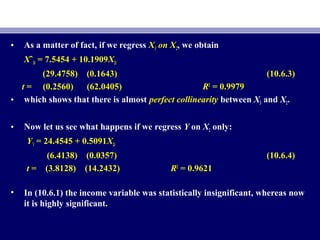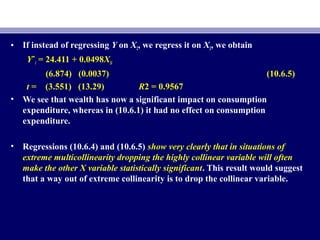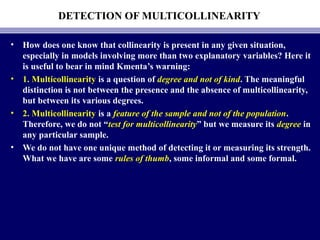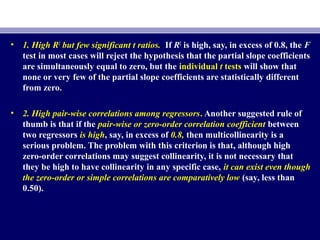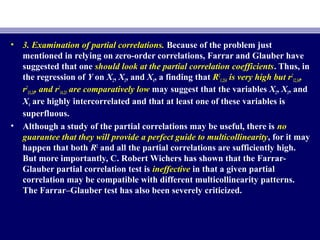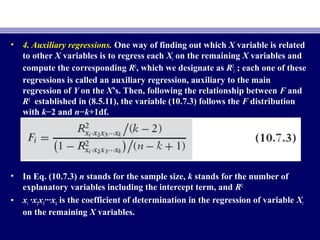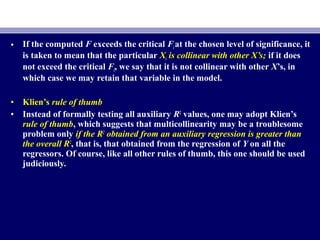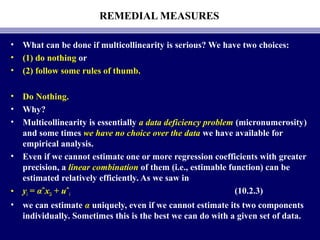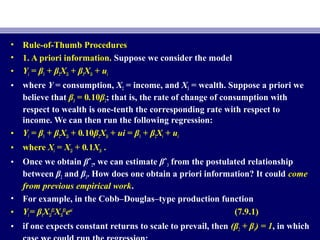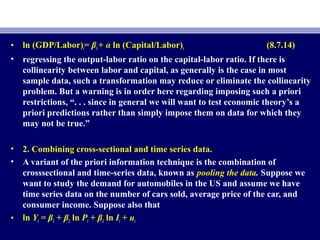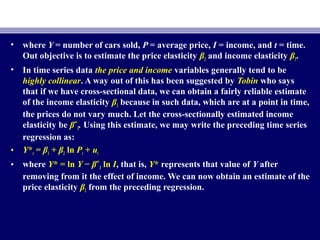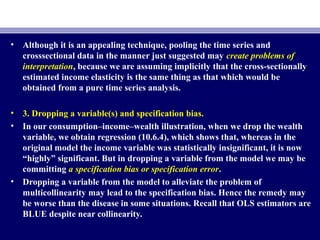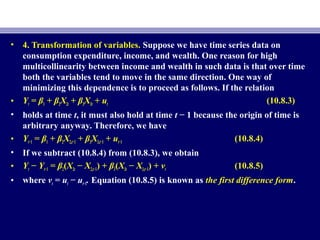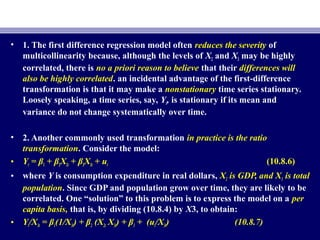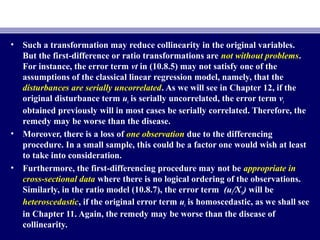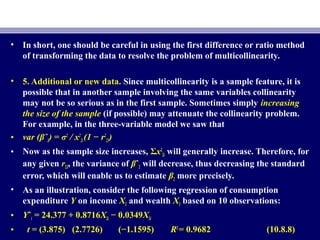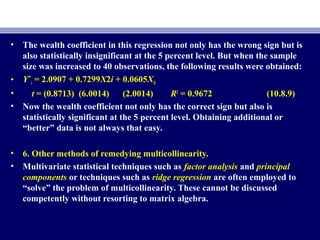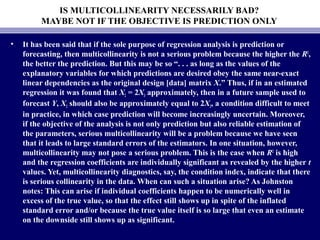1 sur 44

### Econometrics ch11

• 1. 405 ECONOMETRICS Chapter # 11: MULTICOLLINEARITY: WHAT HAPPENS IF THE REGRESSORS ARE CORRELATED? Domodar N. Gujarati Prof. M. El-SakkaProf. M. El-Sakka Dept of Economics Kuwait UniversityDept of Economics Kuwait University
• 2. • In this chapter we take a critical look at this assumption by seeking answers to the following questions: • 1. What is the nature of multicollinearity? • 2. Is multicollinearity really a problem? • 3. What are its practical consequences? • 4. How does one detect it? • 5. What remedial measures can be taken to alleviate the problem of multicollinearity?
• 3. THE NATURE OF MULTICOLLINEARITY • Multicollinearity originally it meant the existence of a “perfect,” or exact, linear relationship among some or all explanatory variables of a regression model. For the k-variable regression involving explanatory variable X1, X2, . . . , Xk (where X1 = 1 for all observations to allow for the intercept term), an exact linear relationship is said to exist if the following condition is satisfied: • λ1X1 + λ2X2 +· · ·+λkXk = 0 (10.1.1) • where λ1, λ2, . . . , λk are constants such that not all of them are zero simultaneously. • Today, however, the term multicollinearity is used to include the case where the X variables are intercorrelated but not perfectly so, as follows: • λ1X1 + λ2X2 +· · ·+λ2Xk + vi = 0 (10.1.2) • where vi is a stochastic error term.
• 4. • To see the difference between perfect and less than perfect multicollinearity, assume, for example, that λ2 ≠ 0. Then, (10.1.1) can be written as: • which shows how X2 is exactly linearly related to other variables. In this situation, the coefficient of correlation between the variable X2 and the linear combination on the right side of (10.1.3) is bound to be unity. • Similarly, if λ2 ≠ 0, Eq. (10.1.2) can be written as: • which shows that X2 is not an exact linear combination of other X’s because it is also determined by the stochastic error term vi.
• 5. • As a numerical example, consider the following hypothetical data: • X2 X3 X*3 • 10 50 52 • 15 75 75 • 18 90 97 • 24 120 129 • 30 150 152 • It is apparent that X3i = 5X2i . Therefore, there is perfect collinearity between X2 and X3 since the coefficient of correlation r23 is unity. The variable X*3 was created from X3 by simply adding to it the following numbers, which were taken from a table of random numbers: 2, 0, 7, 9, 2. Now there is no longer perfect collinearity between X2 and X*3. (X3i = 5X2i + vi ) However, the two variables are highly correlated because calculations will show that the coefficient of correlation between them is 0.9959.
• 6. • The preceding algebraic approach to multicollinearity can be portrayed in Figure 10.1). In this figure the circles Y, X2, and X3 represent, respectively, the variations in Y (the dependent variable) and X2 and X3 (the explanatory variables). The degree of collinearity can be measured by the extent of the overlap (shaded area) of the X2and X3 circles. In the extreme, if X2 and X3 were to overlap completely (or if X2 were completely inside X3, or vice versa), collinearity would be perfect.
• 8. • In passing, note that multicollinearity, as we have defined it, refers only to linear relationships among the X variables. It does not rule out nonlinear relationships among them. For example, consider the following regression model: • Yi = β0 + β1Xi + β2X2 i + β3X3 i + ui (10.1.5) • where, say, Y = total cost of production and X = output. The variables X2 i (output squared) and X3 i (output cubed) are obviously functionally related to Xi, but the relationship is nonlinear. • Why does the classical linear regression model assume that there is no multicollinearity among the X’s? The reasoning is this: • If multicollinearity is perfect, the regression coefficients of the X variables are indeterminate and their standard errors are infinite. • If multicollinearity is less than perfect, the regression coefficients, although determinate, possess large standard errors which means the coefficients cannot be estimated with great precision or accuracy.
• 9. • There are several sources of multicollinearity. • 1. The data collection method employed, for example, sampling over a limited range of the values taken by the regressors in the population. • 2. Constraints on the model or in the population being sampled. For example, in the regression of electricity consumption on income (X2) and house size (X3) (High X2 always mean high X3). • 3. Model specification, for example, adding polynomial terms to a regression model, especially when the range of the X variable is small. • 4. An overdetermined model. This happens when the model has more explanatory variables than the number of observations. • An additional reason for multicollinearity, especially in time series data, may be that the regressors included in the model share a common trend, that is, they all increase or decrease over time.
• 10. ESTIMATION IN THE PRESENCE OF PERFECT MULTICOLLINEARITY • In the case of perfect multicollinearity regression coefficients remain indeterminate and their standard errors are infinite. This fact can be demonstrated readily in terms of the three-variable regression model. Using the deviation form, we can write the three variable regression model as • yi= βˆ2x2i + βˆ3x3i +uˆi (10.2.1) • Now from Chapter 7 we obtain • Assume that X3i = λX2i , where λ is a nonzero constant (e.g., 2, 4, 1.8, etc.). Substituting this into (7.4.7), we obtain
• 11. • which is an indeterminate expression. We can also verify that βˆ3 is indeterminate. • Why do we obtain the result shown in (10.2.2)? Recall the meaning of βˆ2: • It gives the rate of change in the average value of Y as X2 changes by a unit, holding X3 constant. But if X3 and X2 are perfectly collinear, there is no way X3 can be kept constant: As X2 changes, so does X3 by the factor λ. What it means, then, is that there is no way of disentangling the separate influences of X2 and X3 from the given sample.
• 12. • To see this differently, let us substitute X3i = λX2i into (10.2.1) and obtain the following [see also (7.1.9)]: • yi = βˆ2x2i + βˆ3(λx2i)+uˆi • = (βˆ2 + λβˆ3)x2i +uˆi (10.2.3) • = αˆx2i + uˆi • where • αˆ = (βˆ2 + λβˆ3) (10.2.4) • Applying the usual OLS formula to (10.2.3), we get • αˆ = (βˆ2 + λβˆ3) = Σx2i yi/Σx2 2i (10.2.5) • Therefore, although we can estimate α uniquely, there is no way to estimate β2 and β3 uniquely; mathematically • αˆ = βˆ2 + λβˆ3 (10.2.6) • gives us only one equation in two unknowns (note λ is given) and there is an infinity of solutions to (10.2.6) for given values of αˆ and λ.
• 13. • To put this idea in concrete terms, let αˆ = 0.8 and λ = 2. Then we have • 0.8 = βˆ2 + 2βˆ3 (10.2.7) • or • βˆ2 = 0.8 − 2βˆ3 (10.2.8) • Now choose a value of βˆ3 arbitrarily, and we will have a solution for βˆ2. Choose another value for βˆ3, and we will have another solution for βˆ2. No matter how hard we try, there is no unique value for βˆ2. That is in the case of perfect multicollinearity one cannot get a unique solution for the individual regression coefficients. • But notice that one can get a unique solution for linear combinations of these coefficients. The linear combination (β2 + λβ3) is uniquely estimated by α, given the value of λ. In passing, note that in the case of perfect multicollinearity the variances and standard errors of βˆ2 and βˆ3 individually are infinite.
• 14. ESTIMATION IN THE PRESENCE OF “HIGH” BUT “IMPERFECT” MULTICOLLINEARITY • Generally, there is no exact linear relationship among the X variables. Thus, turning to the three-variable model in the deviation form given in (10.2.1), instead of exact multicollinearity, we may have • x3i = λx2i + vi (10.3.1) • where λ ≠ 0 and where vi is a stochastic error term such that x2ivi = 0. • In this case, estimation of regression coefficients β2 and β3 may be possible. For example, substituting (10.3.1) into (7.4.7), we obtain • where use is made of Σx2ivi = 0. A similar expression can be derived for βˆ3. • Now, unlike (10.2.2), there is no reason to believe a priori that (10.3.2) cannot be estimated. Of course, if vi is sufficiently small, say, very close to zero, (10.3.1) will indicate almost perfect collinearity and we shall be back to the indeterminate case of (10.2.2).
• 15. PRACTICAL CONSEQUENCES OF MULTICOLLINEARITY • In cases of near or high multicollinearity, one is likely to encounter the following consequences: • 1. Although BLUE, the OLS estimators have large variances and covariances, making precise estimation difficult. • 2. Because of consequence 1, the confidence intervals tend to be much wider, leading to the acceptance of the “zero null hypothesis” (i.e., the true population coefficient is zero) more readily. • 3. Also because of consequence 1, the t ratio of one or more coefficients tends to be statistically insignificant. • 4. Although the t ratio of one or more coefficients is statistically insignificant, R2 can be very high. • 5. The OLS estimators and their standard errors can be sensitive to small changes in the data. The preceding consequences can be demonstrated as follows.
• 16. • Large Variances and Covariances of OLS Estimators • To see large variances and covariances, recall that for the model (10.2.1) the variances and covariances of βˆ2 and βˆ3 are given by • It is apparent from (7.4.12) and (7.4.15) that as r23 tends toward 1, that is, as collinearity increases, the variances of the two estimators increase and in the limit when r23 = 1, they are infinite. It is equally clear from (7.4.17) that as r23 increases toward 1, the covariance of the two estimators also increases in absolute value.
• 17. • Wider Confidence Intervals • Because of the large standard errors, the confidence intervals for the relevant population parameters tend to be larger, as can be seen from Table 10.2. For example, when r23 = 0.95, the confidence interval for β2 is larger than when r23= 0 by a factor of or about 3. • Therefore, in cases of high multicollinearity, the sample data may be compatible with a diverse set of hypotheses. Hence, the probability of accepting a false hypothesis (i.e., type II error) increases.
• 19. • “Insignificant” t Ratios • We have seen, in cases of high collinearity the estimated standard errors increase dramatically, thereby making the t values smaller. Therefore, in such cases, one will increasingly accept the null hypothesis that the relevant true population value is zero. • A High R2 but Few Significant t Ratios • Consider the k-variable linear regression model: • Yi = β1 + β2X2i + β3X3i +· · ·+βkXki + ui • In cases of high collinearity, it is possible to find that one or more of the partial slope coefficients are individually statistically insignificant on the basis of the t test. Yet the R2 in such situations may be so high, say, in excess of 0.9, that on the basis of the F test one can convincingly reject the hypothesis that β2 = β3 = · · · = βk = 0. Indeed, this is one of the signals of multicollinearity—insignificant t values but a high overall R2 (and a significant F value)!
• 20. • Sensitivity of OLS Estimators and Their Standard Errors to Small Changes in Data • As long as multicollinearity is not perfect, estimation of the regression coefficients is possible but the estimates and their standard errors become very sensitive to even the slightest change in the data. • To see this, consider Table 10.3. Based on these data, we obtain the following multiple regression: Yˆi = 1.1939 + 0.4463X2i + 0.0030X3i (0.7737) (0.1848) (0.0851) t = (1.5431) (2.4151) (0.0358) (10.5.6) R2 = 0.8101 r23 = 0.5523 • cov (βˆ2, βˆ3) = −0.00868 df = 2
• 22. • Regression (10.5.6) shows that none of the regression coefficients is individually significant at the conventional 1 or 5 percent levels of significance, although βˆ2 is significant at the 10 percent level on the basis of a one-tail t test. • Using the data of Table 10.4, we now obtain: Yˆi = 1.2108 + 0.4014X2i + 0.0270X3i (0.7480) (0.2721) (0.1252) t = (1.6187) (1.4752) (0.2158) (10.5.7) R2 = 0.8143 r23 = 0.8285 cov (βˆ2, βˆ3) = −0.0282 df = 2 • As a result of a slight change in the data, we see that βˆ2, which was statistically significant before at the 10 percent level of significance, is no longer significant. Also note that in (10.5.6) cov (βˆ2, βˆ3) = −0.00868 whereas in (10.5.7) it is −0.0282, a more than threefold increase. All these changes may be attributable to increased multicollinearity: In (10.5.6) r23 = 0.5523, whereas in (10.5.7) it is 0.8285. Similarly, the standard errors of βˆ2 and βˆ3 increase between the two regressions, a usual symptom of collinearity.
• 24. AN ILLUSTRATIVE EXAMPLE: CONSUMPTION EXPENDITURE IN RELATION TO INCOME AND WEALTH • Let us reconsider the consumption–income example in table 10.5. we obtain the following regression: Yˆi = 24.7747 + 0.9415X2i − 0.0424X3i (6.7525) (0.8229) (0.0807) t = (3.6690) (1.1442) (−0.5261) (10.6.1) R2 = 0.9635 R¯2 = 0.9531 df = 7 • Regression (10.6.1) shows that income and wealth together explain about 96 percent of the variation in consumption expenditure, and yet neither of the slope coefficients is individually statistically significant. The wealth variable has the wrong sign. Although βˆ2 and βˆ3 are individually statistically insignificant, if we test the hypothesis that β2 = β3 = 0 simultaneously, this hypothesis can be rejected, as Table 10.6 shows.
• 25. Source of variation SS df MSS Due to regression 8,565.5541 2 4,282.7770 Due to residual 324.4459 7 46.3494 • Under the usual assumption we obtain: • F =4282.7770 / 46.3494 = 92.4019 (10.6.2) • This F value is obviously highly significant. Our example shows dramatically what multicollinearity does. • The fact that the F test is significant but the t values of X2 and X3 are individually insignificant means that the two variables are so highly correlated that it is impossible to isolate the individual impact of either income or wealth on consumption.
• 26. • As a matter of fact, if we regress X3 on X2, we obtain Xˆ3i = 7.5454 + 10.1909X2i (29.4758) (0.1643) (10.6.3) t = (0.2560) (62.0405) R2 = 0.9979 • which shows that there is almost perfect collinearity between X3 and X2. • Now let us see what happens if we regress Y on X2 only: Yˆi = 24.4545 + 0.5091X2i (6.4138) (0.0357) (10.6.4) t = (3.8128) (14.2432) R2 = 0.9621 • In (10.6.1) the income variable was statistically insignificant, whereas now it is highly significant.
• 27. • If instead of regressing Y on X2, we regress it on X3, we obtain Yˆi = 24.411 + 0.0498X3i (6.874) (0.0037) (10.6.5) t = (3.551) (13.29) R2 = 0.9567 • We see that wealth has now a significant impact on consumption expenditure, whereas in (10.6.1) it had no effect on consumption expenditure. • Regressions (10.6.4) and (10.6.5) show very clearly that in situations of extreme multicollinearity dropping the highly collinear variable will often make the other X variable statistically significant. This result would suggest that a way out of extreme collinearity is to drop the collinear variable.
• 28. DETECTION OF MULTICOLLINEARITY • How does one know that collinearity is present in any given situation, especially in models involving more than two explanatory variables? Here it is useful to bear in mind Kmenta’s warning: • 1. Multicollinearity is a question of degree and not of kind. The meaningful distinction is not between the presence and the absence of multicollinearity, but between its various degrees. • 2. Multicollinearity is a feature of the sample and not of the population. Therefore, we do not “test for multicollinearity” but we measure its degree in any particular sample. • We do not have one unique method of detecting it or measuring its strength. What we have are some rules of thumb, some informal and some formal.
• 29. • 1. High R2 but few significant t ratios. If R2 is high, say, in excess of 0.8, the F test in most cases will reject the hypothesis that the partial slope coefficients are simultaneously equal to zero, but the individual t tests will show that none or very few of the partial slope coefficients are statistically different from zero. • 2. High pair-wise correlations among regressors. Another suggested rule of thumb is that if the pair-wise or zero-order correlation coefficient between two regressors is high, say, in excess of 0.8, then multicollinearity is a serious problem. The problem with this criterion is that, although high zero-order correlations may suggest collinearity, it is not necessary that they be high to have collinearity in any specific case, it can exist even though the zero-order or simple correlations are comparatively low (say, less than 0.50).
• 30. • 3. Examination of partial correlations. Because of the problem just mentioned in relying on zero-order correlations, Farrar and Glauber have suggested that one should look at the partial correlation coefficients. Thus, in the regression of Y on X2, X3, and X4, a finding that R2 1.234 is very high but r2 12.34, r2 13.24, and r2 14.23 are comparatively low may suggest that the variables X2, X3, and X4 are highly intercorrelated and that at least one of these variables is superfluous. • Although a study of the partial correlations may be useful, there is no guarantee that they will provide a perfect guide to multicollinearity, for it may happen that both R2 and all the partial correlations are sufficiently high. But more importantly, C. Robert Wichers has shown that the Farrar- Glauber partial correlation test is ineffective in that a given partial correlation may be compatible with different multicollinearity patterns. The Farrar–Glauber test has also been severely criticized.
• 31. • 4. Auxiliary regressions. One way of finding out which X variable is related to other X variables is to regress each Xi on the remaining X variables and compute the corresponding R2 , which we designate as R2 i ; each one of these regressions is called an auxiliary regression, auxiliary to the main regression of Y on the X’s. Then, following the relationship between F and R2 established in (8.5.11), the variable (10.7.3) follows the F distribution with k−2 and n−k+1df. • In Eq. (10.7.3) n stands for the sample size, k stands for the number of explanatory variables including the intercept term, and R2 • xi ·x2x3···xk is the coefficient of determination in the regression of variable Xi on the remaining X variables.
• 32. • If the computed F exceeds the critical Fiat the chosen level of significance, it is taken to mean that the particular Xi is collinear with other X’s; if it does not exceed the critical Fi, we say that it is not collinear with other X’s, in which case we may retain that variable in the model. • Klien’s rule of thumb • Instead of formally testing all auxiliary R2 values, one may adopt Klien’s rule of thumb, which suggests that multicollinearity may be a troublesome problem only if the R2 obtained from an auxiliary regression is greater than the overall R2 , that is, that obtained from the regression of Y on all the regressors. Of course, like all other rules of thumb, this one should be used judiciously.
• 33. REMEDIAL MEASURES • What can be done if multicollinearity is serious? We have two choices: • (1) do nothing or • (2) follow some rules of thumb. • Do Nothing. • Why? • Multicollinearity is essentially a data deficiency problem (micronumerosity) and some times we have no choice over the data we have available for empirical analysis. • Even if we cannot estimate one or more regression coefficients with greater precision, a linear combination of them (i.e., estimable function) can be estimated relatively efficiently. As we saw in • yi = αˆx2i + uˆi (10.2.3) • we can estimate α uniquely, even if we cannot estimate its two components individually. Sometimes this is the best we can do with a given set of data.
• 34. • Rule-of-Thumb Procedures • 1. A priori information. Suppose we consider the model • Yi = β1 + β2X2i + β3X3i + ui • where Y = consumption, X2 = income, and X3 = wealth. Suppose a priori we believe that β3 = 0.10β2; that is, the rate of change of consumption with respect to wealth is one-tenth the corresponding rate with respect to income. We can then run the following regression: • Yi = β1 + β2X2i + 0.10β2X3i + ui = β1 + β2Xi + ui • where Xi = X2i + 0.1X3i . • Once we obtain βˆ2, we can estimate βˆ3 from the postulated relationship between β2 and β3. How does one obtain a priori information? It could come from previous empirical work. • For example, in the Cobb–Douglas–type production function • Yi= β1X2i β2 X3i β3 eui (7.9.1) • if one expects constant returns to scale to prevail, then (β2 + β3) = 1, in which
• 35. • ln (GDP/Labor)t= β1+ α ln (Capital/Labor)t (8.7.14) • regressing the output-labor ratio on the capital-labor ratio. If there is collinearity between labor and capital, as generally is the case in most sample data, such a transformation may reduce or eliminate the collinearity problem. But a warning is in order here regarding imposing such a priori restrictions, “. . . since in general we will want to test economic theory’s a priori predictions rather than simply impose them on data for which they may not be true.” • 2. Combining cross-sectional and time series data. • A variant of the priori information technique is the combination of crosssectional and time-series data, known as pooling the data. Suppose we want to study the demand for automobiles in the US and assume we have time series data on the number of cars sold, average price of the car, and consumer income. Suppose also that • ln Yt = β1 + β2 ln Pt + β3 ln It + ut
• 36. • where Y = number of cars sold, P = average price, I = income, and t = time. Out objective is to estimate the price elasticity β2 and income elasticity β3. • In time series data the price and income variables generally tend to be highly collinear. A way out of this has been suggested by Tobin who says that if we have cross-sectional data, we can obtain a fairly reliable estimate of the income elasticity β3 because in such data, which are at a point in time, the prices do not vary much. Let the cross-sectionally estimated income elasticity be βˆ3. Using this estimate, we may write the preceding time series regression as: • Y*t = β1 + β2 ln Pt + ut • where Y* = ln Y − βˆ3 ln I, that is, Y* represents that value of Y after removing from it the effect of income. We can now obtain an estimate of the price elasticity β2 from the preceding regression.
• 37. • Although it is an appealing technique, pooling the time series and crosssectional data in the manner just suggested may create problems of interpretation, because we are assuming implicitly that the cross-sectionally estimated income elasticity is the same thing as that which would be obtained from a pure time series analysis. • 3. Dropping a variable(s) and specification bias. • In our consumption–income–wealth illustration, when we drop the wealth variable, we obtain regression (10.6.4), which shows that, whereas in the original model the income variable was statistically insignificant, it is now “highly” significant. But in dropping a variable from the model we may be committing a specification bias or specification error. • Dropping a variable from the model to alleviate the problem of multicollinearity may lead to the specification bias. Hence the remedy may be worse than the disease in some situations. Recall that OLS estimators are BLUE despite near collinearity.
• 38. • 4. Transformation of variables. Suppose we have time series data on consumption expenditure, income, and wealth. One reason for high multicollinearity between income and wealth in such data is that over time both the variables tend to move in the same direction. One way of minimizing this dependence is to proceed as follows. If the relation • Yt = β1 + β2X2t + β3X3t + ut (10.8.3) • holds at time t, it must also hold at time t − 1 because the origin of time is arbitrary anyway. Therefore, we have • Yt−1 = β1 + β2X2,t−1 + β3X3,t−1 + ut−1 (10.8.4) • If we subtract (10.8.4) from (10.8.3), we obtain • Yt − Yt−1 = β2(X2t − X2,t−1) + β3(X3t − X3,t−1) + vt (10.8.5) • where vt = ut − ut−1. Equation (10.8.5) is known as the first difference form.
• 39. • 1. The first difference regression model often reduces the severity of multicollinearity because, although the levels of X2 and X3 may be highly correlated, there is no a priori reason to believe that their differences will also be highly correlated. an incidental advantage of the first-difference transformation is that it may make a nonstationary time series stationary. Loosely speaking, a time series, say, Yt, is stationary if its mean and variance do not change systematically over time. • 2. Another commonly used transformation in practice is the ratio transformation. Consider the model: • Yt = β1 + β2X2t + β3X3t + ut (10.8.6) • where Y is consumption expenditure in real dollars, X2 is GDP, and X3 is total population. Since GDP and population grow over time, they are likely to be correlated. One “solution” to this problem is to express the model on a per capita basis, that is, by dividing (10.8.4) by X3, to obtain: • Yt/X3t = β1(1/X3t) + β2 (X2t X3t) + β3 + (ut/X3t) (10.8.7)
• 40. • Such a transformation may reduce collinearity in the original variables. But the first-difference or ratio transformations are not without problems. For instance, the error term vt in (10.8.5) may not satisfy one of the assumptions of the classical linear regression model, namely, that the disturbances are serially uncorrelated. As we will see in Chapter 12, if the original disturbance term ut is serially uncorrelated, the error term vt obtained previously will in most cases be serially correlated. Therefore, the remedy may be worse than the disease. • Moreover, there is a loss of one observation due to the differencing procedure. In a small sample, this could be a factor one would wish at least to take into consideration. • Furthermore, the first-differencing procedure may not be appropriate in cross-sectional data where there is no logical ordering of the observations. Similarly, in the ratio model (10.8.7), the error term (ut/X3t) will be heteroscedastic, if the original error term ut is homoscedastic, as we shall see in Chapter 11. Again, the remedy may be worse than the disease of collinearity.
• 41. • In short, one should be careful in using the first difference or ratio method of transforming the data to resolve the problem of multicollinearity. • 5. Additional or new data. Since multicollinearity is a sample feature, it is possible that in another sample involving the same variables collinearity may not be so serious as in the first sample. Sometimes simply increasing the size of the sample (if possible) may attenuate the collinearity problem. For example, in the three-variable model we saw that • var (βˆ2) = σ2 / x2 2i(1 − r2 23) • Now as the sample size increases, Σx2 2i will generally increase. Therefore, for any given r23, the variance of βˆ2 will decrease, thus decreasing the standard error, which will enable us to estimate β2 more precisely. • As an illustration, consider the following regression of consumption expenditure Y on income X2 and wealth X3 based on 10 observations: • Yˆi = 24.377 + 0.8716X2i − 0.0349X3i • t = (3.875) (2.7726) (−1.1595) R2 = 0.9682 (10.8.8)
• 42. • The wealth coefficient in this regression not only has the wrong sign but is also statistically insignificant at the 5 percent level. But when the sample size was increased to 40 observations, the following results were obtained: • Yˆi = 2.0907 + 0.7299X2i + 0.0605X3i • t = (0.8713) (6.0014) (2.0014) R2 = 0.9672 (10.8.9) • Now the wealth coefficient not only has the correct sign but also is statistically significant at the 5 percent level. Obtaining additional or “better” data is not always that easy. • 6. Other methods of remedying multicollinearity. • Multivariate statistical techniques such as factor analysis and principal components or techniques such as ridge regression are often employed to “solve” the problem of multicollinearity. These cannot be discussed competently without resorting to matrix algebra.
• 43. IS MULTICOLLINEARITY NECESSARILY BAD? MAYBE NOT IF THE OBJECTIVE IS PREDICTION ONLY • It has been said that if the sole purpose of regression analysis is prediction or forecasting, then multicollinearity is not a serious problem because the higher the R2 , the better the prediction. But this may be so “. . . as long as the values of the explanatory variables for which predictions are desired obey the same near-exact linear dependencies as the original design [data] matrix X.” Thus, if in an estimated regression it was found that X2 = 2X3 approximately, then in a future sample used to forecast Y, X2 should also be approximately equal to 2X3, a condition difficult to meet in practice, in which case prediction will become increasingly uncertain. Moreover, if the objective of the analysis is not only prediction but also reliable estimation of the parameters, serious multicollinearity will be a problem because we have seen that it leads to large standard errors of the estimators. In one situation, however, multicollinearity may not pose a serious problem. This is the case when R2 is high and the regression coefficients are individually significant as revealed by the higher t values. Yet, multicollinearity diagnostics, say, the condition index, indicate that there is serious collinearity in the data. When can such a situation arise? As Johnston notes: This can arise if individual coefficients happen to be numerically well in excess of the true value, so that the effect still shows up in spite of the inflated standard error and/or because the true value itself is so large that even an estimate on the downside still shows up as significant.